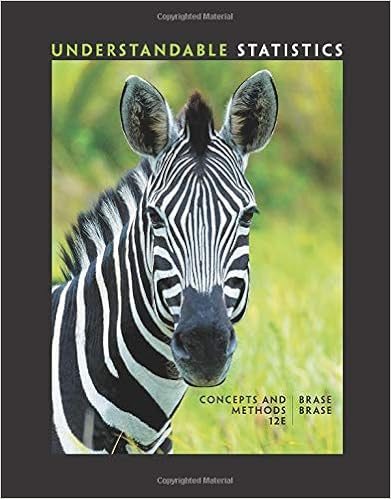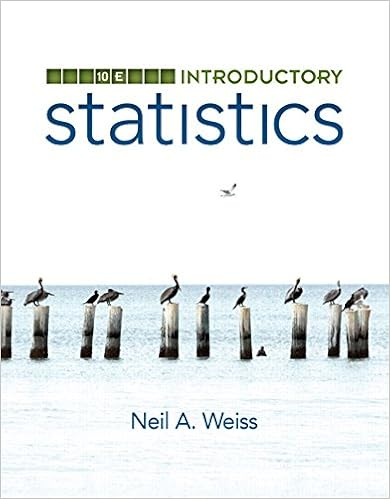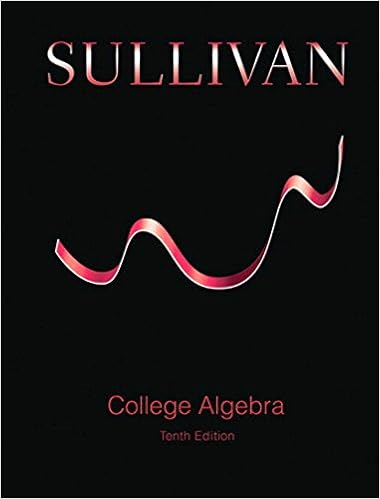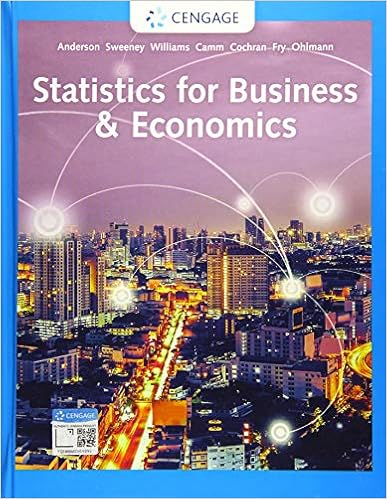# Our solution to that example suggests a general

• Notes
• 341
• 50% (6) 3 out of 6 people found this document helpful

Course Hero uses AI to attempt to automatically extract content from documents to surface to you and others so you can study better, e.g., in search results, to enrich docs, and more. This preview shows page 335 - 339 out of 341 pages.

Our solution to that example suggests a general formula for solving problems of this form:g1842(g1827|g1828) =g1842(g1827uni0009g1853g1866g1856uni0009g1828)g1842(g1828)[our expression for a conditional probability]g1842(g1827|g1828) =g1842(g1827) ⋅g1842(g1828|g1827)g1842(g1828)[by the General Multiplication Rule]g1842(g1827|g1828) =g1842(g1827) ⋅g1842(g1828|g1827)g1842(g1827) ⋅g1842(g1828|g1827) +g1842(g1866g1867g1872uni0009g1827) ⋅g1842(g1828|g1866g1867g1872uni0009g1827)[by the Law of Total Probability]The fact that P(A | B) equals the latter expression is known as Bayes' Rule, or Bayes' Theorem.Probability Trees (3 of 3)Learning ObjectivesUse probability trees as a tool for finding probabilities.Here is a more practical example:
##### We have textbook solutions for you!The document you are viewing contains questions related to this textbook.
Chapter 2 / Exercise 19
Understandable Statistics: Concepts and Methods
BraseExpert VerifiedBrowse all Textbook Solutions
EXAMPLEPolygraph (lie-detector) tests are often routinely administered to employees or prospective employees in sensitivepositions. A National Research Council study in 2002, headed by Stephen Fienberg from CMU, found that lie detectorresults are "better than chance, but well below perfection." Typically, the test may conclude someone is a spy 80% ofthe time when he or she actually is a spy, but 16% of the time the test will conclude someone is a spy when he or she isnot.Let us assume that 1 in 1,000, or .001, of the employees in a certain highly classified workplace are actual spies.LetSbe the event of being a spy, andDbe the event of the polygraph detecting the employee to be a spy.Let's first express the information using probability notations involving events S and D.We are given:* 1 in 1,000, or .001, of the employees are actual spies. →P(S) = .001* the test may conclude someone is a spy 80% of the time when he or she actually is a spy →P(D | S) = .80* 16% of the time, the test will conclude someone is a spy when he or she is not -->P(D | not S) = .16(a) Let's create a tree diagram for this problem, starting, as usual, with the event for which a non-conditional probabilityis given, S. It also makes sense that we start with S, since the natural order is that first a person becomes a spy, and thenhe/she is either detected or not.Note that marked in red are the probabilities that are given, and the rest are completed using the Complement Rule asexplained before.(b) What is the probability that a randomly chosen employee is not a spy, and the test does not detect the employee asone? In other words what is P(not S and not D)?
P(not S and not D) = P(not S) * P(not D | not S) = .999 * .84 = .83916(c) What is the probability that a randomly chosen employeeisa spy, and the test doesnotdetect the employee as one?[This would be an incorrect conclusion.] In other words, what is P(S and not D)P(S and not D) = P(S) * P(not D | S) = .001 * .20 =.0002(d) Suppose the polygraph detects a spy; are you convinced that the employee is actually a spy? Find the probability ofan employee actually being a spy, given that the test claims he or she is. In other words, find P(S | D).Applying Bayes' Rule, we haveP(S | D) = P(S) * P(D | S) / [P(S) * P(D | S) + P(not S) * P(D | not S)]=.001 * .80 / [.001 * .80 + .999 * .16] = .0008/.16064 = .005
The study's conclusion, that more accurate tests than the traditional polygraph are sorely needed, is supported by ouranswer to part (d): if someone is detected as being a spy, the probability is only .005, or half of one percent, that he orshe actually is one.

Course Hero member to access this document

Course Hero member to access this document

End of preview. Want to read all 341 pages?

Course Hero member to access this document

Term
Winter
Professor
N/A
Tags
Mean
##### We have textbook solutions for you!
The document you are viewing contains questions related to this textbook.The document you are viewing contains questions related to this textbook.
Chapter 2 / Exercise 19
Understandable Statistics: Concepts and Methods
BraseExpert Verified
•••